# Design and Simulate Monopulse Tracking System

This example shows how to model and simulate a monopulse tracking system with a transmitter antenna, receiver antenna, and comparator. The monopulse principle is used in many RADAR applications to estimate the position of the target in space by calculating the error in the azimuth and elevation direction. Monopulse tracking can be achieved using various antenna and comparator configurations. The antenna can be a 3-D antenna such as a horn or a planar antenna such as a simple patch antenna.

The comparator can be designed using four rat-race couplers that provide sum, azimuth difference, and elevation difference. Different configurations can be used based on the requirements, but the integration of the antenna and coupler needs to be perfect to achieve the desired results. Integrating the antenna and comparator directly on the hardware results in amplitude and phase mismatches, which can can cause the outputs to vary. Hence, it is important to simulate the integrated model to get accurate results, anticipate the mismatches in phase, and to devise a corrective action before the hardware is fabricated.

### Monopulse System Theory

The monopulse tracking radar can be used to track single or multiple targets by estimating the position of the target in space. This is a diagrammatic representation of the monopulse system.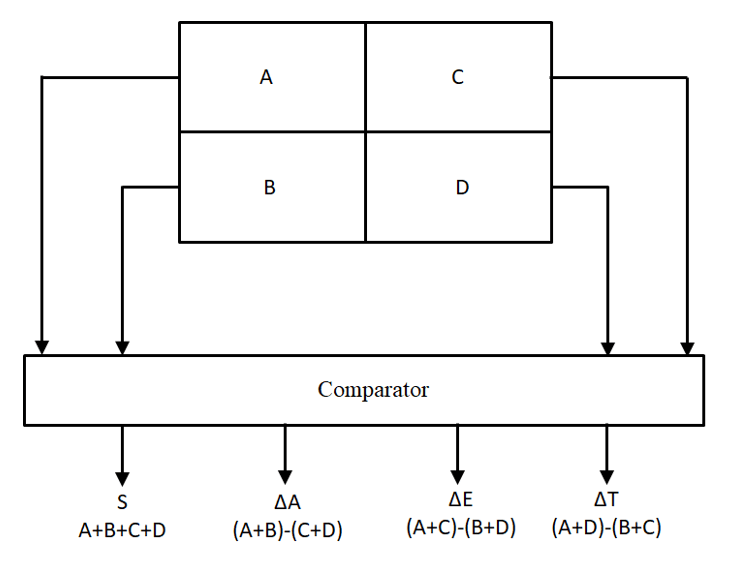Different types of antennas are used to implement the monopulse technique and the diagram shows that the power received from the four quadrants of antenna is given to the passive comparator network to obtain sum (S), azimuth difference (AD), elevation difference (ED), and a cross-quadrant difference (CQD) , all calculated using the following equations.

• (A + B + C + D) = S

• (A + B) – (C + D) = AD

• (A + D) – (B + C) = ED

• (A + C) – (B + D) = CQD

A, B, C, and D are the four quadrants of the 2-by-2 rectangular array antenna. Three receiver channels are required to process these outputs and the target range and position can be estimated using a single pulse. Hence, this technique is named monopulse. The difference signals AD and ED are used for calculating the angular error of the target with respect to the boresight. At the boresight, the sum and difference pattern have a maximum and minimum value, respectively, and this null depth defines the angular resolution and target tracking accuracy. The null depth can be increased by improving the design of the comparator . The combined simulation of the antenna and the comparator is important as it gives the sum and difference patterns that are essential in calculating the monopulse error signal.

### Subsystem Simulation Model

The monopulse system model is built for a transmitter and receiver antenna with four elements arranged in a 2-by-2 rectangular array configuration. The antenna pattern for the transmitter and receiver is designed at the frequency of 2 GHz. The maximum value is obtained for [azimuth elevation] of [0 90] degrees and the gain is 14.8 dBi.

```ant = patchMicrostrip; ant=design(ant,2e9); array = pcbStack(design(rectangularArray,2e9,ant)); figure,mesh(array,'MaxEdgeLength',8e-3); figure,pattern(array,2e9); ```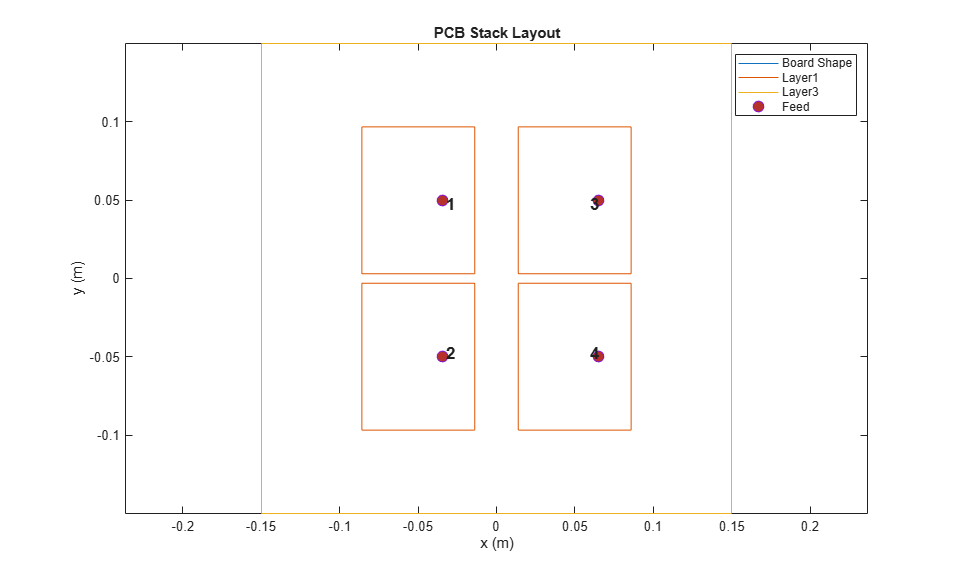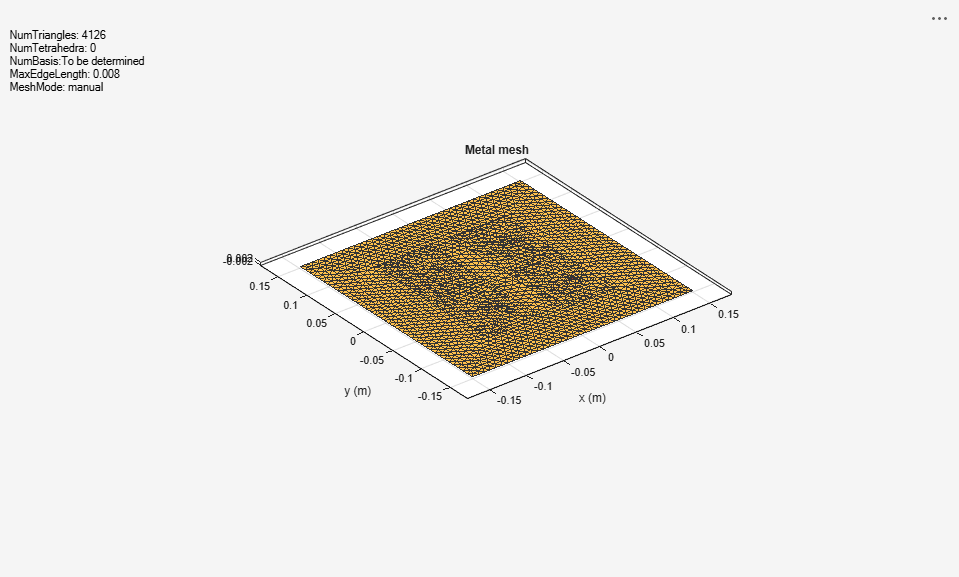Use the 2-by-2 rectangular array antenna at the receiver, but tilt it by 180 degrees so that the two antennas face each other. The calculated pattern has a maximum value of [0 -90] degrees.

```ant = patchMicrostrip; ant = design(ant,2e9); array1 = pcbStack(design(rectangularArray,2e9,ant)); array1.Tilt = 180; figure,mesh(array1,'MaxEdgeLength',8e-3); figure,pattern(array1,2e9); ```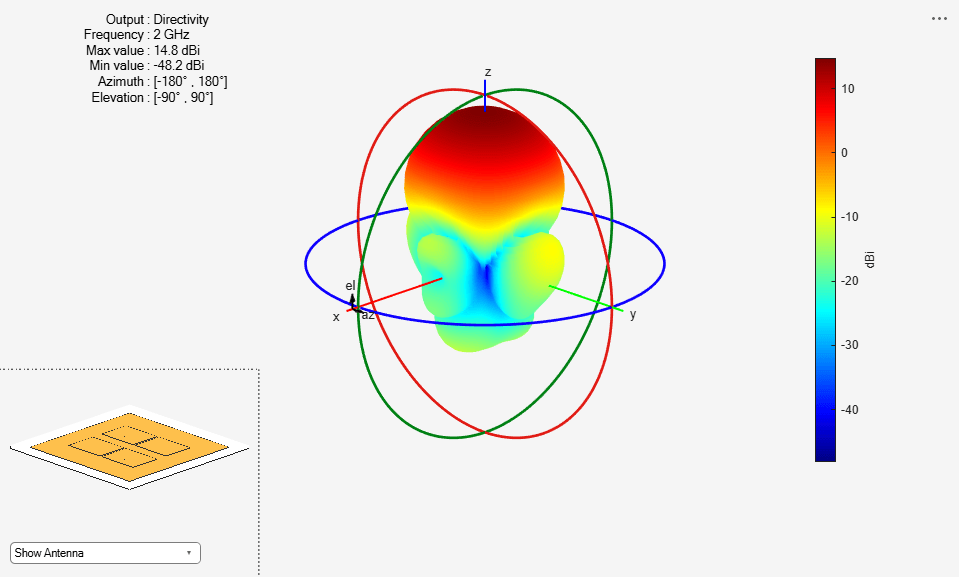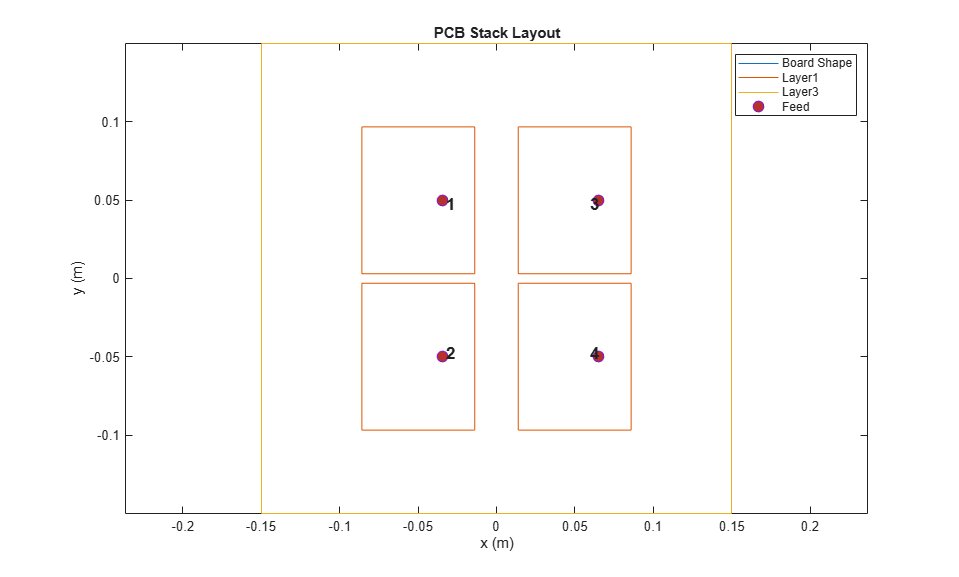The antennas are solved using the method of moments full-wave simulator. The antenna object is given as an input to the transmitter and receiver antenna block in the system model.

### System Simulation Model

The simulation model contains a transmitter and a receiver. A 10 dBm input is divided into four equal parts using the Wilkinson splitter blocks and provided to the array block. The antenna array block in RF Blockset is used to specify the transmitter and receiver antenna. An antenna array object and the direction of departure can be specified in the transmitter block, and the array object and direction of arrival can be specified in the receive array block. The receiver antenna is connected to the comparator circuit, built using rat-race couplers, and the outputs obtained are sum, azimuth difference, and elevation difference. The Wilkinson and the rat-race blocks are modelled using the ideal S-parameters.

The power received in these channels with different angles of arrival on the receive antenna simulates the monopulse tracking system. The antennas in the catalog can be used in the transmit or receive antenna block. The S-parameters of different antennas and comparators can be imported into the model to verify whether these devices are suitable for a monopulse application.

The model can be further refined using S-parameters, calculated using a behavioral model or a method-of-moments solution. These S-parameters can be imported into the model in RF Blockset™ to simulate the monopulse system. The RF Blockset uses the harmonic balance and circuit envelope techniques and takes nonlinear effects into the simulation.

The sum port provides the value of 39.02 dBm, which matches the expected result obtained by adding input power, gain of the transmitter, and receiver antennas. The difference between the sum port and azimuth difference provides a null depth value that is 20 dB lower than the sum signal. The null depth value needs to be improved for better performance of the monopulse system. The observed lower null depth value is caused by a small phase difference between the antenna ports. To compensate for this phase shift, the phaseShift blocks are connected to the output of the antenna ports. The null depth improves and is 44 dB lower than the sum signal.

### Simulation Results

Open and simulate the monopulse tracking system model.

```open_system("monopulse.slx") sim("monopulse.slx"); ```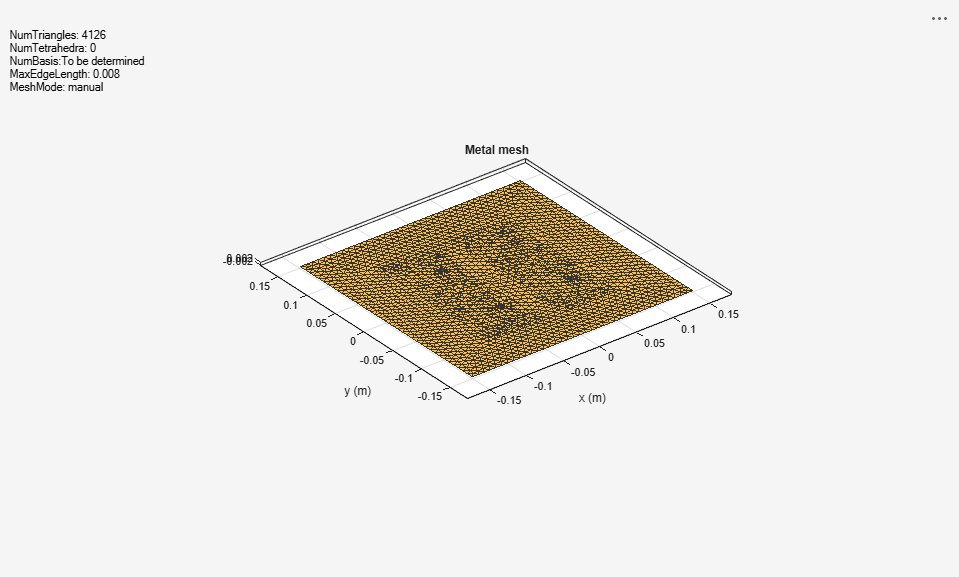The working of the monopulse tracking system can be shown by varying the direction of the target and then measuring the results on the sum port, azimuth, and elevation difference ports. To visualize the system's behavior based on the target location, the simulations were run on this model by varying the angle of arrival on the receiver array block. The angle of arrival specifies the direction from which the signal is received and mimics the behavior of the target's location. The angle of arrival is initially specified along the boresight direction of the receiver antenna at [0 –90], and then decreased in the steps of 10 degrees to measure the power received in the sum and azimuth difference ports.

As the angle of arrival is decreased, the power received on the azimuth difference port increases, and for –70 degrees, this value is greater than the sum port, which signifies that the target is not in the boresight direction. By looking at the relative ratios between the three received powers, the angular position of the receiver can be measured in real time. A similar approach can be used to measure the error observed in the elevation difference port. When the signal arrives from a target at [45 –70], both the azimuth and elevation differences show the change in the values, and both values are close to 29 dB, whereas the sum is around 32 dB.

The model is simulated for all the angles of arrival and the power received in the sum and difference ports is plotted. The figure shows that the elevation difference has a greater null depth compared to the azimuth difference. The elevation difference is formed by subtracting two difference signals from two rat-race couplers, whereas the azimuth difference is formed by subtracting two sum signals from two rat-race couplers. Hence, the null depth for the elevation difference is more than the azimuth difference.

```openfig("result.fig"); ```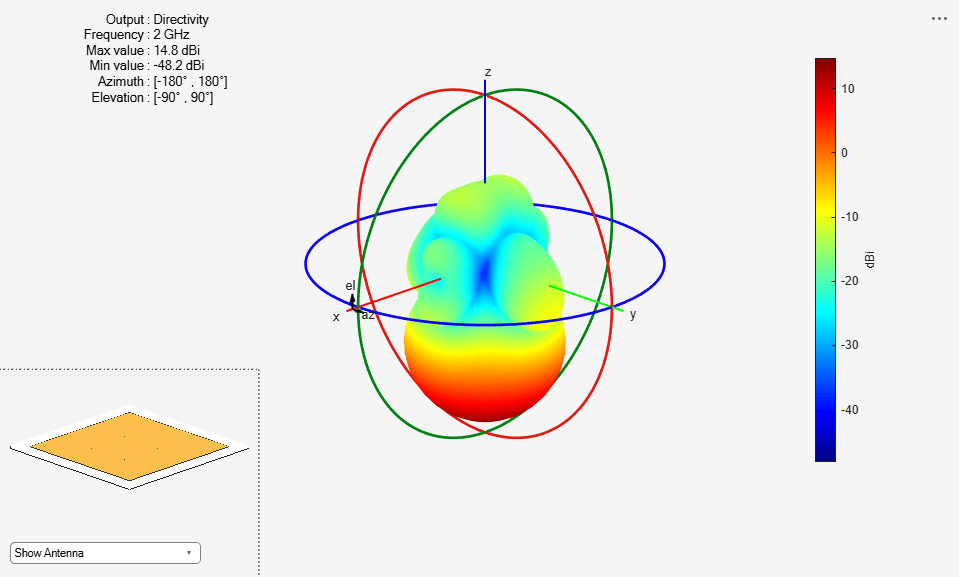### Design Rat-Race Coupler and Wilkinson Power Dividers Using RF PCB Toolbox

Instead of using the ideal models from RF Blockset, more realistic PCB designs can be built and simulated using the EM analysis to reflect a more practical result. The rat-race coupler and the Wilkinson power dividers can be designed and simulated using RF PCB Toolbox™ objects.The S-parameters can be saved in s3p and s4p files, respectively.

Run these commands at the command line to retrace the coupler for the comparator.

```c = couplerRatrace; c = design(c,2e9); spar_ratrace = sparameters(c,linspace(1.5e9,2.5e9,51)); ```

Run these commands at the command line to retrace the Wilkinson splitter for the comparator

```d = wilkinsonSplitter; d = design(d,2e9); spar_wilkinson = sparameters(d,linspace(1.5e9,2.5e9,51)); ```

Run these commands at the command line to create the S3P and S4P files.

```rfwrite(spar_wilkinson,'spar_wilkinson.s3p'); rfwrite(spar_ratrace,'spar_ratrace.s4p'); ```

The Wilkinson and the rat-race coupler blocks are replaced with the S-parameters blocks and the S3P and S4P files calculated above are used.

Open and simulate monopulse system designed with the rat-race coupler and Wilkinson splitter.

```open_system("monopulse_sparameters.slx") sim("monopulse_sparameters.slx"); ```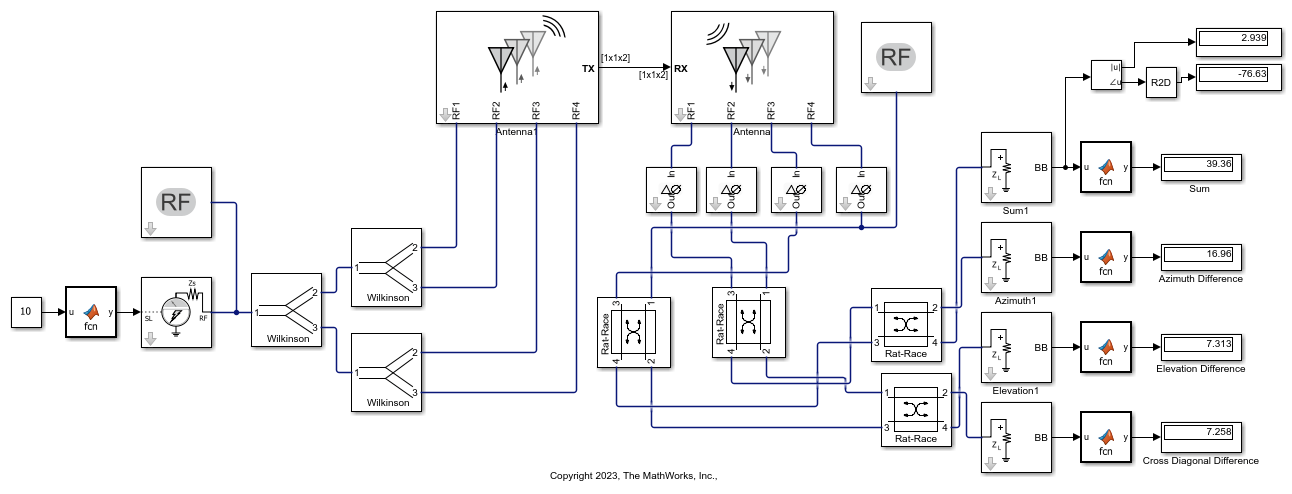The result shows that the null depth is particularly good when the behavioral S-parameters are used, but when the method-of-moments solution is used on the coupler object, the null depth degrades and is 20 dB lower than the sum signal, which is what we might observe when the design is fabricated. Efficient designs of rat-race couplers that provide good isolation between the ports and exact phase difference between the output ports can improve the null depth.

The result shows that the practical designs need to be improved and the amplitude and phase on each port need to be balanced perfectly to acheive the desired result in the monopulse system. This can be prototyped in the model by adding the phase shift block to the rat-race couplers and adjusting the phase of the output signals such that the elevation and azimuth difference can be improved.